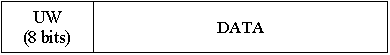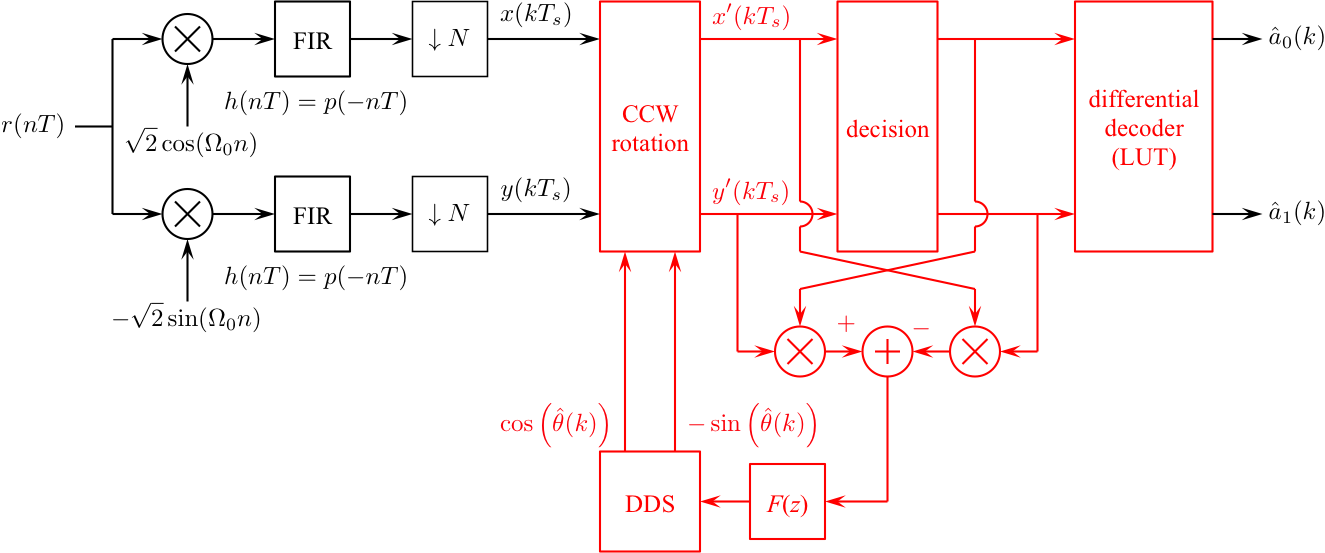# Digital Communications: A Discrete-Time Approachby Michael Rice# Carrier Phase Synchronization for QPSK Using Differential Encoding/Decoding

## Introduction

In the previous QPSK Simulink Exercise the phase of the unmodulated carrier was assumed known. Unfortunately, this is not the case in practice. The carrier phase is unknown and must be extracted from the received signal using a carrier phase recovery subsystem. In this exercise, you will design a PLL-based carrier phase recovery subsystem for QPSK which will be used to process QPSK modulated data contained in the file qpskcrdedata.mat To deal with the 90-degree phase ambiguity of the PLL-based carrier phase synchronizer, this exercise uses differential encoding. In differential encoding, the 2-bit quaternary symbols defined the phase shift of the modulated carrier instead of the absolute phase of the modulated carrier. That is each symbol maps to one of the four possible phase shifts (0, 90, 180, 270) degrees. The detector is based on a conventional coherent QPSK detector followed by a differential decoder. Because the phase ambiguity does not alter the phase shift from one symbol to the next, the decoded symbols are the correct symbols. In this way, differential encoding/decoding is transparent to the 90 degree phase ambiguity.

## Textbook References

M-ary QAM: Section 5.3 (pp. 223 - 240), discrete-time realizations: Section 5.3.2 (pp. 237 - 240), partial response pulse shapes: Section A.2 (pp. 629 - 646), discrete-time PLL: Section C.2 (pp. 380 - 692), carrier phase synchronization for QPSK: Section 7.2 (pp. 338 - 348), differential encoding for phase ambiguity resolution for QPSK: Section 7.7.2 (pp. 373 - 376).

## Specificationsnormalized sample rate: 8 samples/symbol normalized carrier frequency: 0.3 cycles/sample carrier phase: unknown average energy: 2 pulse shape: SRRC (50% excess bandwidth, span = 12 symbols) symbol clock offset: 0 input file qpskcrdedata.mat phase ambiguity resolution differential encoding ```bits phase shift ------------------ 00 0 deg 01 90 deg 10 -90 deg 11 180 deg ``` input message length: 168 symbols (336 bits or 48 ASCII characters) UW 0 0 0 0 0 0 0 0
the packet format is shown belowThe packet is repeated 2 times to allow your PLL to acquire and lock.

## Design the Detector

Design the detector, shown below, using blocks from the Simulink, DSP System, and Communications System Toolboxes. I recommend you start with the detector from the QPSK Carrier Phase Synchronization Simulink Exercise and make changes as necessary.Design the loop filter to create a second order loop to the following specifications: closed-loop equivalent noise bandwidth = 0.025 (normalized to the symbol rate), damping factor = 0.7071.

## Exercise

1. For the detector input, use the From File block and set the Filename to qpskcrdedata.mat and set the sample time to 1.

2. Set the simulation parameters as follows:
 Simulation Time
 Start Time: 0.0 Stop Time: (12+168+4)*8*2
Solver Options
 Type: Fixed-step Solver: discrete (no continuous states) Fixed step size: auto
 Periodic sample time constraint: Unconstrained Tasking mode for periodic sample times: SingleTasking

3. Run the simulation.

4. The detector produces 369 symbol estimates. Even though the UW and DATA fields are repeated twice, the PLL does not lock until part way through the sample sequence.

5. To find the data symbols, look for the 8-bit (4 symbol) synchronization pattern (UW) in the differential decoder output. The 168 symbols following the UW correspond to 48 7-bit ASCII characters.

6. Determine the message using either your Matlab script or an ASCII Table.

7. Plot the phase error (loop filter output) and use this plot to estimate how long (measured in bits) it took your PLL to lock.

8. Plot the eye diagram and signal space projections.
Brigham Young University - Provo | Fulton College of Engineering and Technology | The Church of Jesus Christ of Latter-day Saints